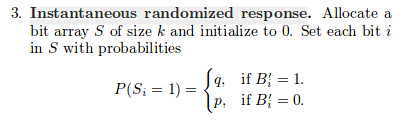# RAPOR privacy enchanced statistics paper: I cannot understand the “Instantaneous randomized response” [closed]

I came across and studies this paper with the title RAPOR privacy enchanced statistics paper. As it mentions the Instantaneous randomized response is calculated from the client as:Does that mean that it i-th bit will be 1 if the the B'i=1 with propability of `q`? And in the end in case of implementation how I will take account the propability `q`?

• You would get a better answer on implementation part at either programming or Math stack exchange. – Limit Apr 10 '18 at 13:16
• As for your first question, it means that if Bi' = 1, there is a probability q such that Si = 1 – Limit Apr 10 '18 at 13:16